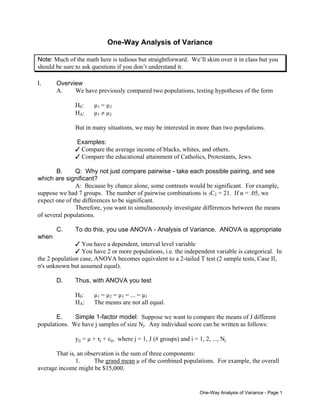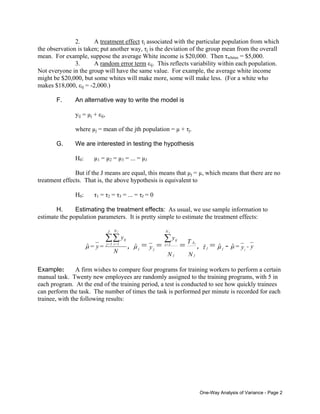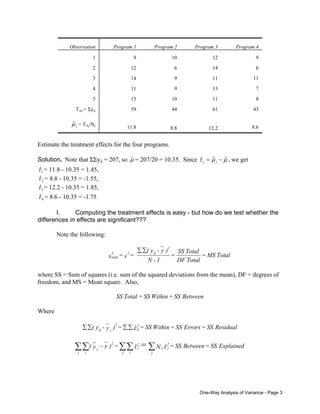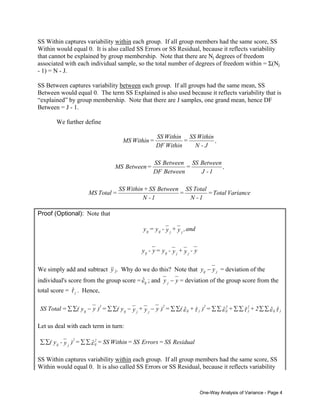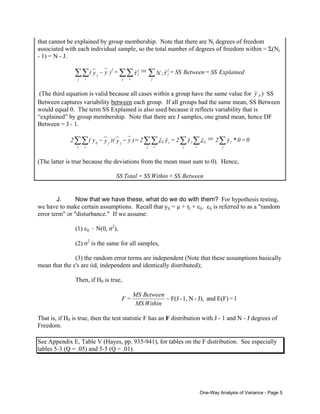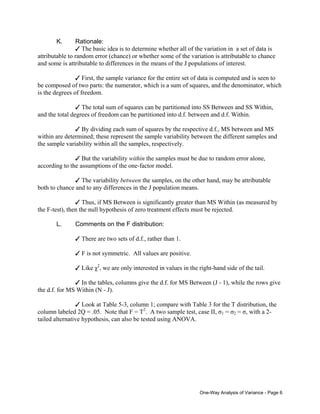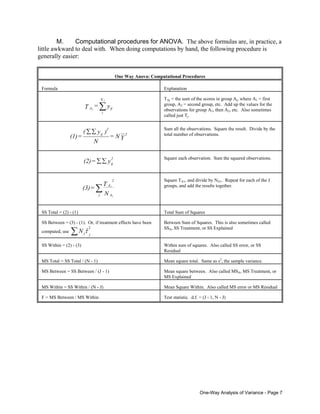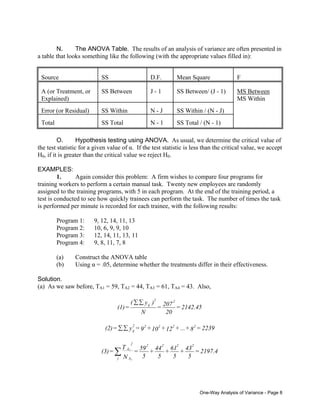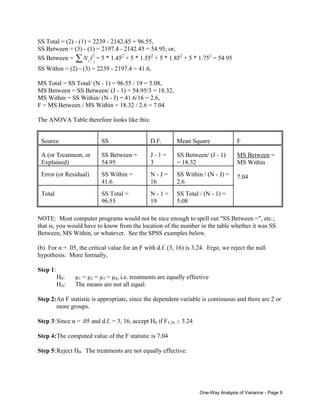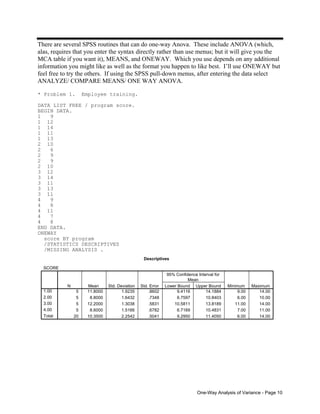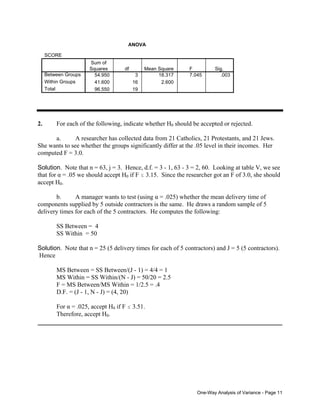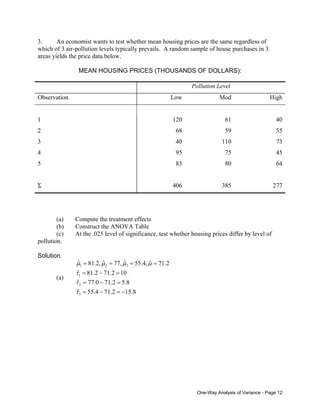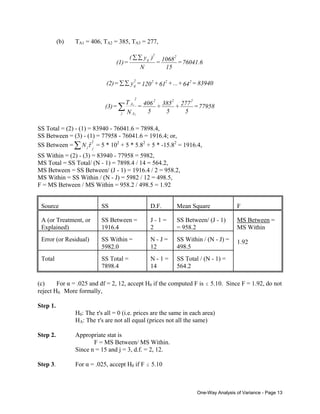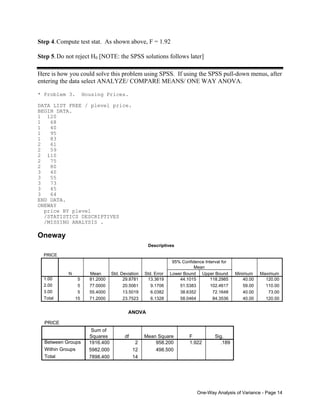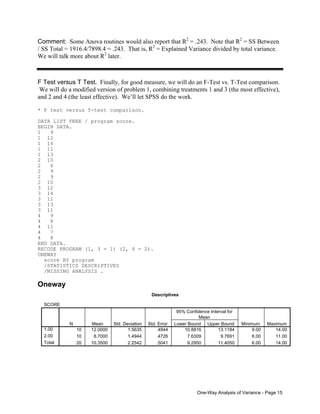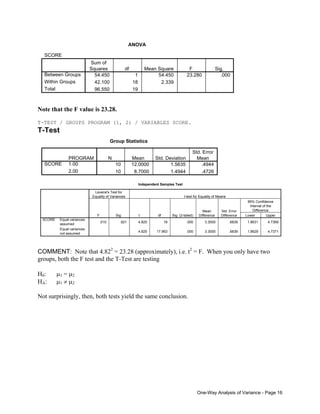Upcoming SlideShare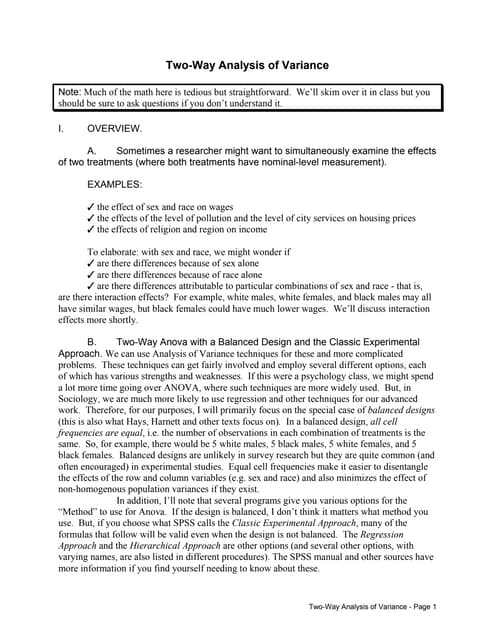X61
1 of 16

### One Way ANOVA.pdf

1. One-Way Analysis of Variance Note: Much of the math here is tedious but straightforward. We’ll skim over it in class but you should be sure to ask questions if you don’t understand it. I. Overview A. We have previously compared two populations, testing hypotheses of the form H0: µ1 = µ2 HA: µ1 ≠ µ2 But in many situations, we may be interested in more than two populations. Examples: T Compare the average income of blacks, whites, and others. T Compare the educational attainment of Catholics, Protestants, Jews. B. Q: Why not just compare pairwise - take each possible pairing, and see which are significant? A: Because by chance alone, some contrasts would be significant. For example, suppose we had 7 groups. The number of pairwise combinations is 7C2 = 21. If α = .05, we expect one of the differences to be significant. Therefore, you want to simultaneously investigate differences between the means of several populations. C. To do this, you use ANOVA - Analysis of Variance. ANOVA is appropriate when T You have a dependent, interval level variable T You have 2 or more populations, i.e. the independent variable is categorical. In the 2 population case, ANOVA becomes equivalent to a 2-tailed T test (2 sample tests, Case II, σ's unknown but assumed equal). D. Thus, with ANOVA you test H0: µ1 = µ2 = µ3 = ... = µJ HA: The means are not all equal. E. Simple 1-factor model: Suppose we want to compare the means of J different populations. We have j samples of size Nj. Any individual score can be written as follows: yij = µ + τj + εij, where j = 1, J (# groups) and i = 1, 2, ..., Nj That is, an observation is the sum of three components: 1. The grand mean µ of the combined populations. For example, the overall average income might be \$15,000. One-Way Analysis of Variance - Page 1
2. 2. A treatment effect τj associated with the particular population from which the observation is taken; put another way, τj is the deviation of the group mean from the overall mean. For example, suppose the average White income is \$20,000. Then τwhites = \$5,000. 3. A random error term εij. This reflects variability within each population. Not everyone in the group will have the same value. For example, the average white income might be \$20,000, but some whites will make more, some will make less. (For a white who makes \$18,000, εij = -2,000.) F. An alternative way to write the model is yij = µj + εij, where µj = mean of the jth population = µ + τj. G. We are interested in testing the hypothesis H0: µ1 = µ2 = µ3 = ... = µJ But if the J means are equal, this means that µj = µ, which means that there are no treatment effects. That is, the above hypothesis is equivalent to H0: τ1 = τ2 = τ3 = ... = τJ = 0 H. Estimating the treatment effects: As usual, we use sample information to estimate the population parameters. It is pretty simple to estimate the treatment effects: y - y = N T N y y N y = y = j j j j - = , = = = , j j j A j ij N 1 = i j j ij N 1 = i J 1 = j µ µ τ µ µ ˆ ˆ ˆ ˆ ˆ ∑ ∑ ∑ Example: A firm wishes to compare four programs for training workers to perform a certain manual task. Twenty new employees are randomly assigned to the training programs, with 5 in each program. At the end of the training period, a test is conducted to see how quickly trainees can perform the task. The number of times the task is performed per minute is recorded for each trainee, with the following results: One-Way Analysis of Variance - Page 2
3. Observation Program 1 Program 2 Program 3 Program 4 1 9 10 12 9 2 12 6 14 8 3 14 9 11 11 4 11 9 13 7 5 13 10 11 8 TAj = Σyij 59 44 61 43 j µ̂ = TAj/Nj 11.8 8.8 12.2 8.6 Estimate the treatment effects for the four programs. Solution. Note that ΣΣyij = 207, so µ̂ = 207/20 = 10.35. Since µ µ τ ˆ ˆ ˆ − = j j , we get 1 ˆ τ = 11.8 - 10.35 = 1.45, 2 ˆ τ = 8.8 - 10.35 = -1.55, 3 ˆ τ = 12.2 - 10.35 = 1.85, 4 ˆ τ = 8.6 - 10.35 = -1.75 I. Computing the treatment effects is easy - but how do we test whether the differences in effects are significant??? Note the following: Total MS = Total DF Total SS = 1 - N ) y - y ( = s = s 2 ij 2 2 total ∑ ∑ where SS = Sum of squares (i.e. sum of the squared deviations from the mean), DF = degrees of freedom, and MS = Mean square. Also, Between SS + Within SS = Total SS Where Residual SS = Errors SS = Within SS = = ) y - y ( 2 ij 2 j ij ε ˆ ∑ ∑ ∑ ∑ Explained SS = Between SS = N = ) y y ( 2 j j j 2 j i j 2 j i j = τ τ ˆ ˆ ∑ ∑ ∑ ∑ ∑ − One-Way Analysis of Variance - Page 3
4. SS Within captures variability within each group. If all group members had the same score, SS Within would equal 0. It is also called SS Errors or SS Residual, because it reflects variability that cannot be explained by group membership. Note that there are Nj degrees of freedom associated with each individual sample, so the total number of degrees of freedom within = Σ(Nj - 1) = N - J. SS Between captures variability between each group. If all groups had the same mean, SS Between would equal 0. The term SS Explained is also used because it reflects variability that is “explained” by group membership. Note that there are J samples, one grand mean, hence DF Between = J - 1. We further define Variance Total = 1 - N Total SS = 1 - N Between SS + Within SS = Total MS , 1 - J Between SS = Between DF Between SS = Between MS , J - N Within SS = Within DF Within SS = Within MS Proof (Optional): Note that y - y + y - y = y - y and , y + y - y = y j j ij ij j j ij ij We simply add and subtract y j. Why do we do this? Note that j ij y y − = deviation of the individual's score from the group score = ij ε ˆ ; and y y j − = deviation of the group score from the total score = j τ ˆ . Hence, τ ε τ ε τ ε ˆ ˆ ˆ ˆ ˆ ˆ j ij 2 j 2 ij 2 j ij 2 j j ij 2 ij 2 + + = ) + ( = ) y y + y y ( = ) y y ( = Total SS ∑ ∑ ∑ ∑ ∑ ∑ ∑ ∑ − − ∑ ∑ − ∑ ∑ Let us deal with each term in turn: Residual SS = Errors SS = Within SS = = ) y - y ( 2 ij 2 j ij ε ˆ ∑ ∑ ∑ ∑ SS Within captures variability within each group. If all group members had the same score, SS Within would equal 0. It is also called SS Errors or SS Residual, because it reflects variability One-Way Analysis of Variance - Page 4
5. that cannot be explained by group membership. Note that there are Nj degrees of freedom associated with each individual sample, so the total number of degrees of freedom within = Σ(Nj - 1) = N - J. Explained SS = Between SS = N = ) y y ( 2 j j j 2 j i j 2 j i j = τ τ ˆ ˆ ∑ ∑ ∑ ∑ ∑ − (The third equation is valid because all cases within a group have the same value for y j.) SS Between captures variability between each group. If all groups had the same mean, SS Between would equal 0. The term SS Explained is also used because it reflects variability that is “explained” by group membership. Note that there are J samples, one grand mean, hence DF Between = J - 1. 0 = 0 * 2 2 = 2 = ) y y )( y y ( = j j ij i j j j ij i j j j ij i j τ ε τ τ ε ˆ ˆ ˆ ˆ ˆ ∑ ∑ ∑ ∑ ∑ ∑ − − ∑ 2 (The latter is true because the deviations from the mean must sum to 0). Hence, Between SS + Within SS = Total SS J. Now that we have these, what do we do with them? For hypothesis testing, e have to make certain assumptions. Recall that yij = µ + τj + εij. εij is referred to as a "random error te or or all samples, pendent (Note that these assumptions basically mean that the ε are iid, independent and identically distributed); w rm" "disturbance." If we assume: (1) εij - N(0, σ2 ), (2) σ2 is the same f (3) the random error terms are inde 's Then, if H0 is true, 1 = E(F) and J), - N 1, - (J F ~ Within MS Between MS = F That is, if H0 is true, then the test statistic F has an F distribution with J - 1 and N - J degrees of Freedom. ix E, Table V (Hayes, pp. 935-941), for tables on the F distribution. See especially See Append tables 5-3 (Q = .05) and 5-5 (Q = .01). One-Way Analysis of Variance - Page 5
6. K. Rationale: T The basic idea is to determine whether all of the variation in a set of data is attribut to chance) or whether some of the variation is attributable to chance and some is att is seen to e composed of two parts: the numerator, which is a sum of squares, and the denominator, which is the degrees o m of squares can be partitioned into SS Between and SS Within, nd the total degrees of freedom can be partitioned into d.f. between and d.f. Within. nd MS ithin are determined; these represent the sample variability between the different samples and the sample var be due to random error alone, ccording to the assumptions of the one-factor model. the other hand, may be attributable oth to chance and to any differences in the J population means. MS Within (as measured by e F-test), then the null hypothesis of zero treatment effects must be rejected. an 1. ve. e right-hand side of the tail. give e d.f. for MS Within (N - J). 5-3, column 1; compare with Table 3 for the T distribution, the olumn labeled 2Q = .05. Note that F = T2 . A two sample test, case II, σ1 = σ2 = σ, with a 2- tailed alternati able random error ( ributable to differences in the means of the J populations of interest. T First, the sample variance for the entire set of data is computed and b f freedom. T The total su a T By dividing each sum of squares by the respective d.f., MS between a w iability within all the samples, respectively. T But the variability within the samples must a T The variability between the samples, on b T Thus, if MS Between is significantly greater than th L. Comments on the F distribution: T There are two sets of d.f., rather th T F is not symmetric. All values are positi T Like χ2 , we are only interested in values in th T In the tables, columns give the d.f. for MS Between (J - 1), while the rows th T Look at Table c ve hypothesis, can also be tested using ANOVA. One-Way Analysis of Variance - Page 6
7. M. Computational procedures for ANOVA. The above formulas are, in practice, a little awkward to deal with. When doing computations by hand, the following procedure is generally easier: One Way Anova: Computational Procedures Formula Explanation y = T ij N i A j j ∑ TAj = the sum of the scores in group Aj, where A1 = first group, A2 = second group, etc. Add up the values for the observations for group A1, then A2, etc. Also sometimes called just Tj. Y N = N ) y ( = (1) 2 2 ij ∑ ∑ Sum all the observations. Square the result. Divide by the total number of observations. y = (2) 2 ij ∑ ∑ Square each observation. Sum the squared observations. N T = (3) A A 2 j j j ∑ Square TA1, and divide by NA1. Repeat for each of the J groups, and add the results together. SS Total = (2) - (1) Total Sum of Squares SS Between = (3) - (1). Or, if treatment effects have been computed, use 2 ˆ j j N ∑ τ Between Sum of Squares. This is also sometimes called SSA, SS Treatment, or SS Explained SS Within = (2) - (3) Within sum of squares. Also called SS error, or SS Residual MS Total = SS Total / (N - 1) Mean square total. Same as s2 , the sample variance. MS Between = SS Between / (J - 1) Mean square between. Also called MSA, MS Treatment, or MS Explained MS Within = SS Within / (N - J) Mean Square Within. Also called MS error or MS Residual F = MS Between / MS Within Test statistic. d.f. = (J - 1, N - J) One-Way Analysis of Variance - Page 7
8. N. The ANOVA Table. The results of an analysis of variance are often presented in a table that looks something like the following (with the appropriate values filled in): Source SS D.F. Mean Square F A (or Treatment, or Explained) SS Between J - 1 SS Between/ (J - 1) Error (or Residual) SS Within N - J SS Within / (N - J) Total SS Total N - 1 SS Total / (N - 1) MS Between MS Within O. Hypothesis testing using ANOVA. As usual, we determine the critical value of the test statistic for a given value of α. If the test statistic is less than the critical value, we accept H0, if it is greater than the critical value we reject H0. EXAMPLES: 1. Again consider this problem: A firm wishes to compare four programs for training workers to perform a certain manual task. Twenty new employees are randomly assigned to the training programs, with 5 in each program. At the end of the training period, a test is conducted to see how quickly trainees can perform the task. The number of times the task is performed per minute is recorded for each trainee, with the following results: Program 1: 9, 12, 14, 11, 13 Program 2: 10, 6, 9, 9, 10 Program 3: 12, 14, 11, 13, 11 Program 4: 9, 8, 11, 7, 8 (a) Construct the ANOVA table (b) Using α = .05, determine whether the treatments differ in their effectiveness. Solution. (a) As we saw before, TA1 = 59, TA2 = 44, TA3 = 61, TA4 = 43. Also, 2142.45 = 20 207 = N ) y ( = (1) 2 2 ij ∑ ∑ 2239 = 8 + ... + 12 + 10 + 9 = y = (2) 2 2 2 2 2 ij ∑ ∑ 2197.4 = 5 43 + 5 61 + 5 44 + 5 59 = N T = (3) 2 2 2 2 A A 2 j j j ∑ One-Way Analysis of Variance - Page 8
9. SS Total = (2) - (1) = 2239 - 2142.45 = 96.55, SS Between = (3) - (1) = 2197.4 - 2142.45 = 54.95; or, SS Between = = 5 * 1.45 2 ˆ j j N ∑ τ 2 + 5 * 1.552 + 5 * 1.852 + 5 * 1.752 = 54.95 SS Within = (2) - (3) = 2239 - 2197.4 = 41.6, MS Total = SS Total/ (N - 1) = 96.55 / 19 = 5.08, MS Between = SS Between/ (J - 1) = 54.95/3 = 18.32, MS Within = SS Within/ (N - J) = 41.6/16 = 2.6, F = MS Between / MS Within = 18.32 / 2.6 = 7.04 The ANOVA Table therefore looks like this: Source SS D.F. Mean Square F A (or Treatment, or Explained) SS Between = 54.95 J - 1 = 3 SS Between/ (J - 1) = 18.32 Error (or Residual) SS Within = 41.6 N - J = 16 SS Within / (N - J) = 2.6 Total SS Total = 96.55 N - 1 = 19 SS Total / (N - 1) = 5.08 MS Between = MS Within 7.04 NOTE: Most computer programs would not be nice enough to spell out "SS Between =", etc.; that is, you would have to know from the location of the number in the table whether it was SS Between, MS Within, or whatever. See the SPSS examples below. (b) For α = .05, the critical value for an F with d.f. (3, 16) is 3.24. Ergo, we reject the null hypothesis. More formally, Step 1: H0: µ1 = µ2 = µ3 = µ4, i.e. treatments are equally effective HA: The means are not all equal. Step 2:An F statistic is appropriate, since the dependent variable is continuous and there are 2 or more groups. Step 3:Since α = .05 and d.f. = 3, 16, accept H0 if F3,16 # 3.24 Step 4:The computed value of the F statistic is 7.04 Step 5:Reject H0. The treatments are not equally effective. One-Way Analysis of Variance - Page 9
10. There are several SPSS routines that can do one-way Anova. These include ANOVA (which, alas, requires that you enter the syntax directly rather than use menus; but it will give you the MCA table if you want it), MEANS, and ONEWAY. Which you use depends on any additional information you might like as well as the format you happen to like best. I’ll use ONEWAY but feel free to try the others. If using the SPSS pull-down menus, after entering the data select ANALYZE/ COMPARE MEANS/ ONE WAY ANOVA. * Problem 1. Employee training. DATA LIST FREE / program score. BEGIN DATA. 1 9 1 12 1 14 1 11 1 13 2 10 2 6 2 9 2 9 2 10 3 12 3 14 3 11 3 13 3 11 4 9 4 8 4 11 4 7 4 8 END DATA. ONEWAY score BY program /STATISTICS DESCRIPTIVES /MISSING ANALYSIS . Descriptives SCORE 5 11.8000 1.9235 .8602 9.4116 14.1884 9.00 14.00 5 8.8000 1.6432 .7348 6.7597 10.8403 6.00 10.00 5 12.2000 1.3038 .5831 10.5811 13.8189 11.00 14.00 5 8.6000 1.5166 .6782 6.7169 10.4831 7.00 11.00 20 10.3500 2.2542 .5041 9.2950 11.4050 6.00 14.00 1.00 2.00 3.00 4.00 Total N Mean Std. Deviation Std. Error Lower Bound Upper Bound 95% Confidence Interval for Mean Minimum Maximum One-Way Analysis of Variance - Page 10
11. ANOVA SCORE 54.950 3 18.317 7.045 .003 41.600 16 2.600 96.550 19 Between Groups Within Groups Total Sum of Squares df Mean Square F Sig. 2. For each of the following, indicate whether H0 should be accepted or rejected. a. A researcher has collected data from 21 Catholics, 21 Protestants, and 21 Jews. She wants to see whether the groups significantly differ at the .05 level in their incomes. Her computed F = 3.0. Solution. Note that n = 63, j = 3. Hence, d.f. = 3 - 1, 63 - 3 = 2, 60. Looking at table V, we see that for α = .05 we should accept H0 if F # 3.15. Since the researcher got an F of 3.0, she should accept H0. b. A manager wants to test (using α = .025) whether the mean delivery time of components supplied by 5 outside contractors is the same. He draws a random sample of 5 delivery times for each of the 5 contractors. He computes the following: SS Between = 4 SS Within = 50 Solution. Note that n = 25 (5 delivery times for each of 5 contractors) and J = 5 (5 contractors). Hence MS Between = SS Between/(J - 1) = 4/4 = 1 MS Within = SS Within/(N - J) = 50/20 = 2.5 F = MS Between/MS Within = 1/2.5 = .4 D.F. = (J - 1, N - J) = (4, 20) For α = .025, accept H0 if F # 3.51. Therefore, accept H0. One-Way Analysis of Variance - Page 11
12. 3. An economist wants to test whether mean housing prices are the same regardless of which of 3 air-pollution levels typically prevails. A random sample of house purchases in 3 areas yields the price data below. MEAN HOUSING PRICES (THOUSANDS OF DOLLARS): Pollution Level Observation Low Mod High 1 120 61 40 2 68 59 55 3 40 110 73 4 95 75 45 5 83 80 64 Σ 406 385 277 (a) Compute the treatment effects (b) Construct the ANOVA Table (c) At the .025 level of significance, test whether housing prices differ by level of pollution. Solution. (a) 8 . 15 2 . 71 4 . 55 ˆ 8 . 5 2 . 71 0 . 77 ˆ 10 2 . 71 2 . 81 ˆ 2 . 71 ˆ , 4 . 55 ˆ , 77 ˆ , 2 . 81 ˆ 3 2 1 3 2 1 − = − = = − = = − = = = = = τ τ τ µ µ µ µ One-Way Analysis of Variance - Page 12
13. (b) TA1 = 406, TA2 = 385, TA3 = 277, 76041.6 = 15 1068 = N ) y ( = (1) 2 2 ij ∑ ∑ 83940 = 64 + ... + 61 + 120 = y = (2) 2 2 2 2 ij ∑ ∑ 77958 = 5 277 + 5 385 + 5 406 = N T = (3) 2 2 2 A A 2 j j j ∑ SS Total = (2) - (1) = 83940 - 76041.6 = 7898.4, SS Between = (3) - (1) = 77958 - 76041.6 = 1916.4; or, SS Between = = 5 * 10 2 ˆ j j N ∑ τ 2 + 5 * 5.82 + 5 * -15.82 = 1916.4, SS Within = (2) - (3) = 83940 - 77958 = 5982, MS Total = SS Total/ (N - 1) = 7898.4 / 14 = 564.2, MS Between = SS Between/ (J - 1) = 1916.4 / 2 = 958.2, MS Within = SS Within / (N - J) = 5982 / 12 = 498.5, F = MS Between / MS Within = 958.2 / 498.5 = 1.92 Source SS D.F. Mean Square F A (or Treatment, or Explained) SS Between = 1916.4 J - 1 = 2 SS Between/ (J - 1) = 958.2 Error (or Residual) SS Within = 5982.0 N - J = 12 SS Within / (N - J) = 498.5 Total SS Total = 7898.4 N - 1 = 14 SS Total / (N - 1) = 564.2 MS Between = MS Within 1.92 (c) For α = .025 and df = 2, 12, accept H0 if the computed F is # 5.10. Since F = 1.92, do not reject H0. More formally, Step 1. H0: The τ's all = 0 (i.e. prices are the same in each area) HA: The τ's are not all equal (prices not all the same) Step 2. Appropriate stat is F = MS Between/ MS Within. Since n = 15 and j = 3, d.f. = 2, 12. Step 3. For α = .025, accept H0 if F # 5.10 One-Way Analysis of Variance - Page 13
14. Step 4.Compute test stat. As shown above, F = 1.92 Step 5.Do not reject H0 [NOTE: the SPSS solutions follows later] Here is how you could solve this problem using SPSS. If using the SPSS pull-down menus, after entering the data select ANALYZE/ COMPARE MEANS/ ONE WAY ANOVA. * Problem 3. Housing Prices. DATA LIST FREE / plevel price. BEGIN DATA. 1 120 1 68 1 40 1 95 1 83 2 61 2 59 2 110 2 75 2 80 3 40 3 55 3 73 3 45 3 64 END DATA. ONEWAY price BY plevel /STATISTICS DESCRIPTIVES /MISSING ANALYSIS . Oneway Descriptives PRICE 5 81.2000 29.8781 13.3619 44.1015 118.2985 40.00 120.00 5 77.0000 20.5061 9.1706 51.5383 102.4617 59.00 110.00 5 55.4000 13.5019 6.0382 38.6352 72.1648 40.00 73.00 15 71.2000 23.7523 6.1328 58.0464 84.3536 40.00 120.00 1.00 2.00 3.00 Total N Mean Std. Deviation Std. Error Lower Bound Upper Bound 95% Confidence Interval for Mean Minimum Maximum ANOVA PRICE 1916.400 2 958.200 1.922 .189 5982.000 12 498.500 7898.400 14 Between Groups Within Groups Total Sum of Squares df Mean Square F Sig. One-Way Analysis of Variance - Page 14
15. Comment: Some Anova routines would also report that R2 = .243. Note that R2 = SS Between / SS Total = 1916.4/7898.4 = .243. That is, R2 = Explained Variance divided by total variance. We will talk more about R2 later. F Test versus T Test. Finally, for good measure, we will do an F-Test vs. T-Test comparison. We will do a modified version of problem 1, combining treatments 1 and 3 (the most effective), and 2 and 4 (the least effective). We’ll let SPSS do the work. * F test versus T-test comparison. DATA LIST FREE / program score. BEGIN DATA. 1 9 1 12 1 14 1 11 1 13 2 10 2 6 2 9 2 9 2 10 3 12 3 14 3 11 3 13 3 11 4 9 4 8 4 11 4 7 4 8 END DATA. RECODE PROGRAM (1, 3 = 1) (2, 4 = 2). ONEWAY score BY program /STATISTICS DESCRIPTIVES /MISSING ANALYSIS . Oneway Descriptives SCORE 10 12.0000 1.5635 .4944 10.8816 13.1184 9.00 14.00 10 8.7000 1.4944 .4726 7.6309 9.7691 6.00 11.00 20 10.3500 2.2542 .5041 9.2950 11.4050 6.00 14.00 1.00 2.00 Total N Mean Std. Deviation Std. Error Lower Bound Upper Bound 95% Confidence Interval for Mean Minimum Maximum One-Way Analysis of Variance - Page 15
16. ANOVA SCORE 54.450 1 54.450 23.280 .000 42.100 18 2.339 96.550 19 Between Groups Within Groups Total Sum of Squares df Mean Square F Sig. Note that the F value is 23.28. T-TEST / GROUPS PROGRAM (1, 2) / VARIABLES SCORE. T-Test Group Statistics 10 12.0000 1.5635 .4944 10 8.7000 1.4944 .4726 PROGRAM 1.00 2.00 SCORE N Mean Std. Deviation Std. Error Mean Independent Samples Test .010 .921 4.825 18 .000 3.3000 .6839 1.8631 4.7369 4.825 17.963 .000 3.3000 .6839 1.8629 4.7371 Equal variances assumed Equal variances not assumed SCORE F Sig. Levene's Test for Equality of Variances t df Sig. (2-tailed) Mean Difference Std. Error Difference Lower Upper 95% Confidence Interval of the Difference t-test for Equality of Means COMMENT: Note that 4.822 = 23.28 (approximately), i.e. t2 = F. When you only have two groups, both the F test and the T-Test are testing H0: µ1 = µ2 HA: µ1 ≠ µ2 Not surprisingly, then, both tests yield the same conclusion. One-Way Analysis of Variance - Page 16